# Chaco-交互式图表

Chaco是一个2D的绘图库，如果你安装了Python(x,y)的话，可以在pythonxy的安装目录下的找到Chaco的demo程序:

```   \pythonxy\doc\Enthought Tool Suite\Chaco\examples\demo.py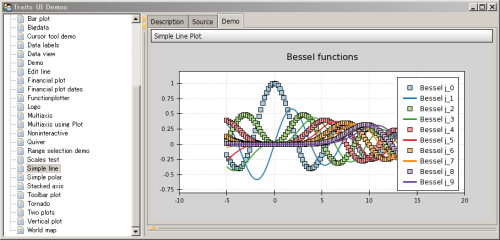Chaco的演示程序的界面，包含了很多例子可以同时查看源程序和运行结果

## 面向脚本绘图

Chaco提供了类似Matlab和pylab的绘图方式，我们称之为面向脚本的绘图方式。下面的程序从enthought.chaco.shell中导入脚本绘图相关的内容，然后用numpy快速产生一个正弦波的x,y坐标的数组，然后传递给plot函数进行绘图:

 ``` 1 2 3 4 5 6 7 8 9 10``` ```import numpy as np from enthought.chaco.shell import * x = np.linspace(-2*np.pi, 2*np.pi, 100) y = np.sin(x) plot(x, y, "r-") title("First plot") ytitle("sin(x)") show() ```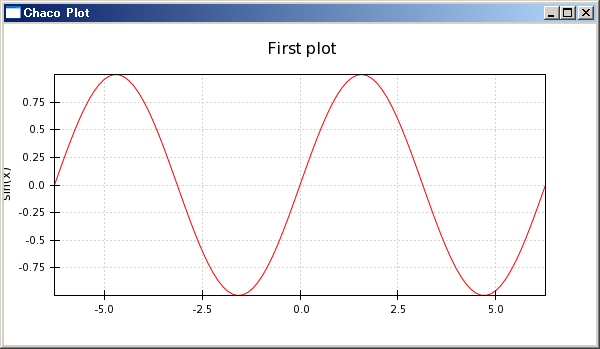plot函数的第三个参数中的"r"指定绘图的颜色为红色，"-"指定绘图的线型为实线。title函数为绘图添加标题，ytitle为Y轴添加标题，show()函数最终显示绘图结果。

## 面向应用绘图

 ``` 1 2 3 4 5 6 7 8 9 10 11 12 13 14 15 16 17 18 19 20 21 22 23 24``` ```from enthought.traits.api import HasTraits, Instance from enthought.traits.ui.api import View, Item from enthought.chaco.api import Plot, ArrayPlotData from enthought.enable.component_editor import ComponentEditor from numpy import linspace, sin class LinePlot(HasTraits): plot = Instance(Plot) traits_view = View( Item('plot',editor=ComponentEditor(), show_label=False), width=500, height=500, resizable=True, title="Chaco Plot") def __init__(self): super(LinePlot, self).__init__() x = linspace(-14, 14, 100) y = sin(x) * x**3 plotdata = ArrayPlotData(x=x, y=y) plot = Plot(plotdata) plot.plot(("x", "y"), type="line", color="blue") plot.title = "sin(x) * x^3" self.plot = plot if __name__ == "__main__": LinePlot().configure_traits() ```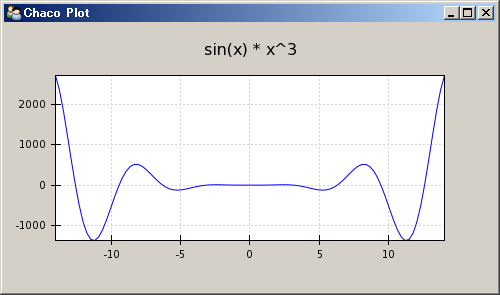• HasTraits, Instance：这两个从traits库中导入，HasTraits是所有拥有Trait属性的类的父类，我们自己定义的LinePlot类继承于它。而Instance用来创建一个Trait属性，此属性的值为某个指定的类的实例。
• View, Item：从traits.ui库导入，View用来创建一个生成用户界面用的视图，而Item则用来定义视图中的元素。
• Plot, ArrayPlotData：从chaco库中导入，Plot本身是一个描述绘图的类，它的祖先类中有HasTraits，因此它本身也是一个拥有trait属性的类，ArrayPlotData是用来统一保存绘图所用的数据的类。也就是说Plot管理绘图，而ArrayPlotData则用来管理绘图所用的数据。
• ComponentEditor：从enable库中导入，用户界面视图中使用ComponentEditor来显示LinePlot类的plot属性。如果trait属性为Int、Str或者Float之类的简单类型的话，系统能够自动的帮我们选择对应的GUI元素来显示它们。但是系统不知道如何显示Chaco中定义的Plot这样的的类型，因此我们必须手工指定采用ComponentEditor来显示Plot。
• linspace, sin：从numpy库中导入，linspace用来产生一个等差数列，numpy中的sin函数可以自动对数组中的每个元素进行计算。

```class LinePlot(HasTraits):
plot = Instance(Plot)
```

```traits_view = View(
Item('plot',editor=ComponentEditor(), show_label=False),
width=500, height=500, resizable=True, title="Chaco Plot")
```

```def __init__(self):
super(LinePlot, self).__init__()
x = linspace(-14, 14, 100)
y = sin(x) * x**3
plotdata = ArrayPlotData(x=x, y=y)
```

```plot = Plot(plotdata)
```

Plot类将Chaco中提供的许多真正用来绘图的对象进行包装，提供了一个统一的接口用来创建和管理这些绘图对象。在今后深入学习Chaco的过程中我们将通过分析Plot类的实现来了解Chaco库的设计思想。

```plot.plot(("x", "y"), type="line", color="blue")
```

```plot.title = "sin(x) * x^3"
self.plot = plot
```

```if __name__ == "__main__":
LinePlot().configure_traits()
```

 ``` 1 2 3 4 5 6 7 8 9 10 11 12 13 14 15 16 17 18 19 20 21 22 23 24 25 26 27 28 29 30 31 32 33``` ```from enthought.traits.api import HasTraits, Instance from enthought.traits.ui.api import View, Item from enthought.chaco.api import Plot, ArrayPlotData, Legend from enthought.enable.component_editor import ComponentEditor from numpy import linspace, sin, cos class LinePlot(HasTraits): plot = Instance(Plot) traits_view = View( Item('plot',editor=ComponentEditor(), show_label=False), width=500, height=500, resizable=True, title="Chaco Plot") def __init__(self): super(LinePlot, self).__init__() x = linspace(-14, 14, 100) y1 = sin(x) * x**3 y2 = cos(x) * x**3 plotdata = ArrayPlotData(x=x, y1=y1, y2=y2) plot = Plot(plotdata) plot.plot(("x", "y1"), type="line", color="blue", name="sin(x) * x**3") plot.plot(("x", "y2"), type="line", color="red", name="cos(x) * x**3") plot.plot(("x", "y2"), type="scatter", color="red", marker = "circle", marker_size = 2, name="cos(x) * x**3 points") plot.title = "Multiple Curves" self.plot = plot legend = Legend(padding=10, align="ur") legend.plots = plot.plots plot.overlays.append(legend) if __name__ == "__main__": lineplot = LinePlot() lineplot.configure_traits() ```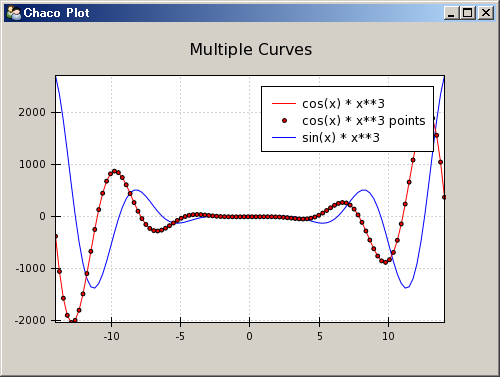```legend = Legend(component=plot, padding=10, align="ur")
legend.plots = plot.plots
plot.overlays.append(legend)
```

```>>> lineplot.plot.plots
{'cos(x) * x**3 points': [<enthought.chaco.scatterplot.ScatterPlot object at 0x1436B4B0>],
'cos(x) * x**3': [<enthought.chaco.lineplot.LinePlot object at 0x1436B120>],
'sin(x) * x**3': [<enthought.chaco.lineplot.LinePlot object at 0x143140F0>]}
```

### 容器(Container)概述

• HPlotContainer：内容横向排列的容器
• VPlotContainer：内容竖向排列的容器
• GridPlotContainer：内容按照网格排列的容器

 ``` 1 2 3 4 5 6 7 8 9 10 11 12 13 14 15 16 17 18 19 20 21 22 23 24``` ```from enthought.traits.api import HasTraits, Instance from enthought.traits.ui.api import View, Item from enthought.chaco.api import HPlotContainer, ArrayPlotData, Plot from enthought.enable.component_editor import ComponentEditor from numpy import linspace, sin class ContainerExample(HasTraits): plot = Instance(HPlotContainer) traits_view = View(Item('plot', editor=ComponentEditor(), show_label=False), width=1000, height=600, resizable=True, title="Chaco Plot") def __init__(self): super(ContainerExample, self).__init__() x = linspace(-14, 14, 100) y = sin(x) * x**3 plotdata = ArrayPlotData(x=x, y=y) scatter = Plot(plotdata) scatter.plot(("x", "y"), type="scatter", color="blue") line = Plot(plotdata) line.plot(("x", "y"), type="line", color="blue") container = HPlotContainer(scatter, line) self.plot = container if __name__ == "__main__": ContainerExample().configure_traits() ```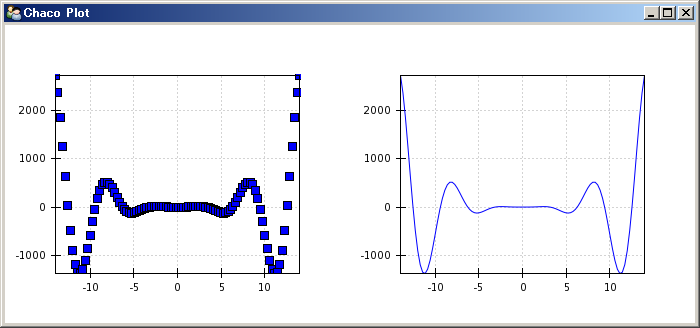```scatter.padding_right = 0
line.y_axis.orientation = "right"
```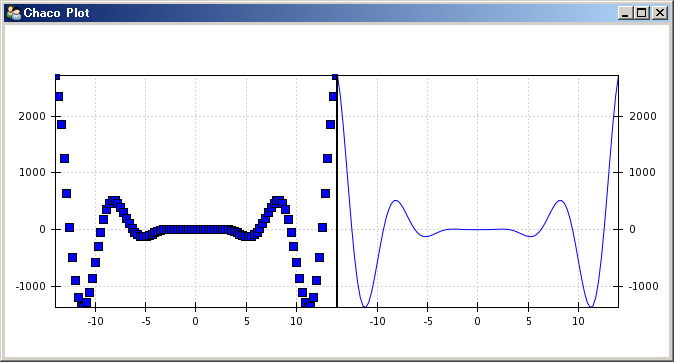### 编辑绘图属性

 ``` 1 2 3 4 5 6 7 8 9 10 11 12 13 14 15 16 17 18 19 20 21 22 23 24 25 26 27 28 29 30 31 32 33 34 35 36 37 38 39 40 41 42``` ```from enthought.traits.api import HasTraits, Instance, Int, Color from enthought.traits.ui.api import View, Group, Item from enthought.enable.component_editor import ComponentEditor from enthought.chaco.api import marker_trait, Plot, ArrayPlotData from numpy import linspace, sin class ScatterPlotTraits(HasTraits): plot = Instance(Plot) color = Color("blue") marker = marker_trait marker_size = Int(4) traits_view = View( Group(Item('color', label="Color"), Item('marker', label="Marker"), Item('marker_size', label="Size"), Item('plot', editor=ComponentEditor(), show_label=False), orientation = "vertical"), width=800, height=600, resizable=True, title="Chaco Plot") def __init__(self): super(ScatterPlotTraits, self).__init__() x = linspace(-14, 14, 100) y = sin(x) * x**3 plotdata = ArrayPlotData(x = x, y = y) plot = Plot(plotdata) self.renderer = plot.plot(("x", "y"), type="scatter", color="blue") self.plot = plot def _color_changed(self): self.renderer.color = self.color def _marker_changed(self): self.renderer.marker = self.marker def _marker_size_changed(self): self.renderer.marker_size = self.marker_size if __name__ == "__main__": ScatterPlotTraits().configure_traits() ```ScatterPlotTraits类定义了4个trait属性，其中一个是我们已经熟知的plot属性，其余的三个分别为color，marker和marker_size。color是一个Color属性，marker_size是一个Int属性，marker比较特别，它是在Chaco的scatter_makers.py中定义的一个Trait属性，采用字典创建，将一个描述点型的字符串映射到点型对应的类，这样我们通过界面上的下拉选择框选择某个点型名称时，在程序内部实际上选择的是其对应的类。